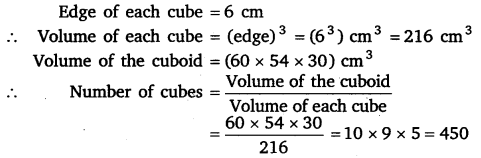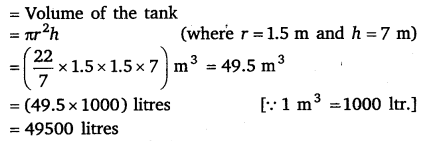# Class 8 Maths NCERT Solutions for Chapter – 11 Mensuration Ex – 11.4

## Mensuration

Question 1.
Given a cylindrical tank, in which situation will you find surface area and in which situation volume.
(a)
To find how much it can hold.
(b) Number of cement bags required to plaster it.
(c) To find the number of smaller tanks that can be filled with water from it.

Solution:
(a) Volume
(b) Surface area
(c) Volume

Question 2.
Diameter of cylinder A is 7 cm, and the height is 14 cm. Diameter of cylinder B is 14 cm and height is 7 cm. Without doing any calculations can you suggest whose volume is greater? Verify it by finding the volume of both the cylinders. Check whether the cylinder with greater volume also has greater surface area?

Solution:

Cylinder B has greater volume.
Surface area of cylinder A
= (2πrh + 2πr2 sq. units,Thus, cylinder having greater volume also has greater surface area.

Question 3.
Find the height of a cuboid whose base area is 180 cm2 and volume is 900 cm3?

Solution:
Volume of the cuboid =900 cm3
⇒ (Area of the base) x Height = 900 cm3
⇒ 180 x Height = 900
⇒ Height =$\frac { 900 }{ 180 }$ = 5
Hence, the height of the cuboid is 5 cm.

Question 4.
A cuboid is of dimensions 60 cm x 54 cm x 30 cm. How many small cubes with side 6 cm can be placed in the given cuboid?

Solution:Question 5.
Find the height of the cylinder whose volume is 1.54 m3 and diameter of the base is 140 cm?

Solution:
Let the h be the height of cylinder whose radius, r =$\frac { 140 }{ 2 }$ cm = 70 cm =$\frac { 70 }{ 100 }$ m and volume =1.54 m3.Question 6.
A milk tank is in the form of cylinder whose radius is 1.5 m and length is 7 m. Find the quantity of milk in liters that can be stored in the tank?

Solution:

Quantity of milk that can be stored in the tank = Volume of the tankQuestion 7.
If each edge of a cube is doubled,
(i)
how many times will its surface area increase?
(ii) how many times will its volume increase?

Solution:
Let x units be the edge of the cube. Then, its surface area = 6x2 and its volume = x3.
When its edge is doubled,
(i) Its surface area = 6 (2x)2 = 6 x 4 x 2 = 24 x 2
⇒ The surface area of the new cube will be 4 times that of the original cube.
(ii) Its volume = (2x)3 = 8x3.
⇒ The volume of the new cube will be 8 times that of the original cube.

Question 8.
Water is pouring into a cuboidal reservoir at the rate of 60 liters per minute. If the volume of reservoir is 108 m3, find the number of hours it will take to fill the reservoir.

Solution:

Volume of the reservoir =108 m3
= 108 x 1000 litres
= 108000 litres
Since water is pouring into reservoir @ 60 litres per minute
∴ Time taken to fill the reservoir =$\frac { 108000 }{ 60\times 60 }$ hours
= 30 hours

### Take Your IIT JEE Coaching to Next Level with Examtube

• Mentoring & Teaching by IITians
• Regular Testing & Analysis
• Preparation for Various Engineering Entrance Exams
• Support for School/Board Exams